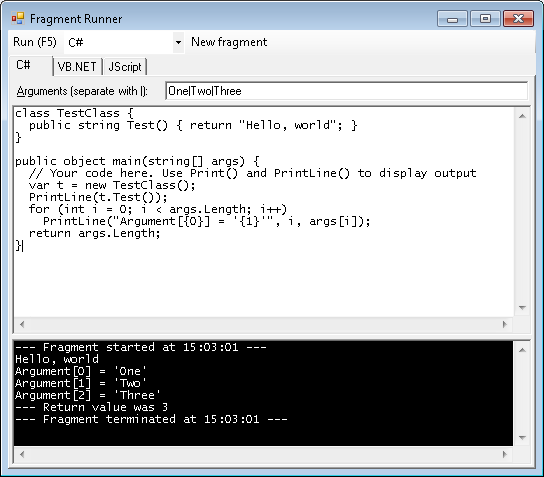You are viewing this site over HTTP, which is not secure. Please consider switching to HTTPS instead.

# Fragment Runner

Fragment Runner actually consists of three parts:

• Fragment Runner - An application to quickly test and run code fragments
• MOBZystems.CodeFragments - A library allowing the compilation of code fragments
• Function Plotter - A demo application showing the use of the CodeFragments library to plot a user-defined function

Together, these allow you to create, test and use code fragments written in C#, VB.NET and Jscript in your own applications.

## Fragment Runner: The Application

Simply select the language you want to use, type in your code fragment and press F5 to run it:The output of your fragment is printed to the pane below. The fragment can call Print() and PrintLine() to display output. Both accept String.Format-like arguments.

## MOBZystems.CodeFragments: The library

Part of the source code is a library enabling you to create in-memory, executable assemblies. You can both capture their console-like output and the return value. The code below compiles the C# fragment

```PrintLine("Executed at " + DateTime.Now.Ticks.ToString());
if (args.Length == 0) return "Nothing to do!";
if (args.Length == 1) return args;
return args.Length;
```

It then calls this fragment (by invoking Run on it) without arguments, with a single argument and with two arguments:

```Sub Main()
' Create a compiler for C#. Also supports VB.NET and JScript
Dim compiler As FragmentCompiler =
FragmentCompiler.CreateCompiler(FragmentCompiler.LanguageTypeEnum.CSharp)
' Compile a source code fragment that returns something based on its arguments
Dim c As CodeFragment = compiler.CompileExpression(
"PrintLine(""Executed at "" + DateTime.Now.Ticks.ToString());" +
"if (args.Length == 0) return ""Nothing to do!"";" +
"if (args.Length == 1) return args;" +
"return args.Length;"
)
' If compiling succeeded, run the fragment three times and display the result
If c.Succeeded Then
' No arguments --> "Nothing to do!"
Console.WriteLine(c.Run())
Console.Write(c.Output)
' A single argument --> "and a one"
Console.WriteLine(c.Run(New String() {"and a one"}))
Console.Write(c.Output)
' More than 1 argument --> "2"
Console.WriteLine(c.Run(New String() {"one", "two"}))
Console.Write(c.Output)
Else
' On errors, dump the first error
Console.Error.WriteLine(c.Errors(0).ErrorText)
End If
End Sub```

The compiled fragment takes a variables number of arguments, which all have to be of type string, and returns an object

## Example: Function Plotter

A MOBZystems.CodeFragments.FragmentCompiler.CodeFragment is also used to produce the following plot in another example program: FuncPlotter.The source code for this form is approx. one printed page, but the core is much shorter:

```'''
''' Create a fragment to calculate values for the function definition in the text box.
''' If it compiles, run it and store the values
'''
Private Sub plotButton_Click(sender As Object, e As EventArgs) Handles plotButton.Click
Dim func As String

Select Case Me.functionTextBox.Text
Case "snor"
func = snor
Case "bult"
func = bult
Case Else
func = Me.functionTextBox.Text
End Select

Dim funcDef As String =
"double xmin = double.Parse(args);" +
"double xmax = double.Parse(args);" +
"int nx = int.Parse(args);" +
"double ymin = double.Parse(args);" +
"double ymax = double.Parse(args);" +
"int ny = int.Parse(args);" +
"double dx = (xmax - xmin) / (nx - 1);" +
"double dy = (ymax - ymin) / (ny - 1);" +
"double[,] values = new double[nx, ny];" +
"double x = xmin;" +
"for (int ix = 0; ix < nx; ix++) {" +
"  double y = ymin;" +
"  for (int iy = 0; iy < ny; iy++) {" +
"    values[ix, iy] = " + func + ";" +
"    y += dy;" +
"  }" +
"  x += dx;" +
"}" +
"return values;"

Dim fragment As CodeFragment = _compiler.CompileExpression(funcDef)

If fragment.Succeeded Then
' Run the function definition and get the result into o
Dim o As Object = fragment.Run(New String() {
CStr(_xMin), CStr(_xMax), CStr(_xSteps),
CStr(_yMin), CStr(_yMax), CStr(_ySteps)
})

' Cast to an array of doubles and store in values()
Me._values = DirectCast(o, Double(,))

' Calculate plotted coordinates, bounding box, etc. so we don't have to when painting
CalculatePlot()

' Make sure we repaint!
Me.plotPictureBox.Invalidate()

Else

MessageBox.Show(Me, fragment.Errors(0).ErrorText, "Error in function definition", MessageBoxButtons.OK, MessageBoxIcon.Error)

End If
End Sub
```

This code evaluates the function of x and y that was typed into the functionTextBox text box by the user for a set of values for x and y. First, a code fragment is constructed in funcDef that doesn't do much more than evaluate the function for the given ranges and stores the result in an array. It then returns the array. (The fun part, of course, is that the fragment is written in C# while the rest of the application is written in VB.NET!)

The fragment is then compiled using the member variable _compiler that was created in the constructor of the form:

```Public Sub New() InitializeComponent()
' Set up a CSharp fragment-compiler
Me._compiler = FragmentCompiler.CreateCompiler(
FragmentCompiler.LanguageTypeEnum.CSharp
)
End Sub
```

If compilation succeeded, the fragment is executed, passing in the range of values we want the function to be evaluated over. The result is used to set up the actual plot.

As you can see, pretty powerful stuff!